# COMPSCI 102 Introduction to Discrete Mathematics Graphs Lecture

• Slides: 43
Download presentation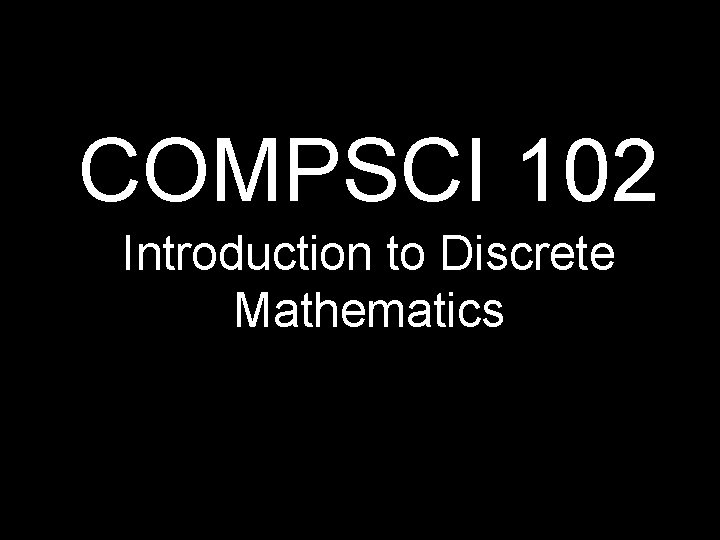COMPSCI 102 Introduction to Discrete Mathematics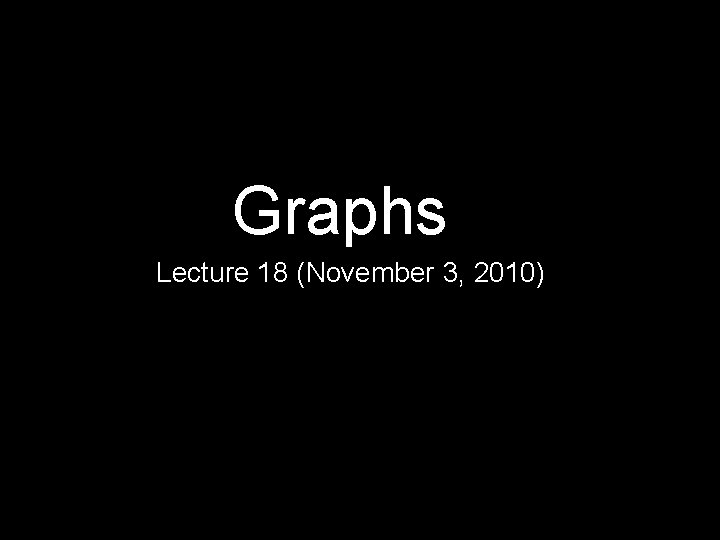Graphs Lecture 18 (November 3, 2010)What’s a tree? A tree is a connected graph with no cyclesTreeNot a. Tree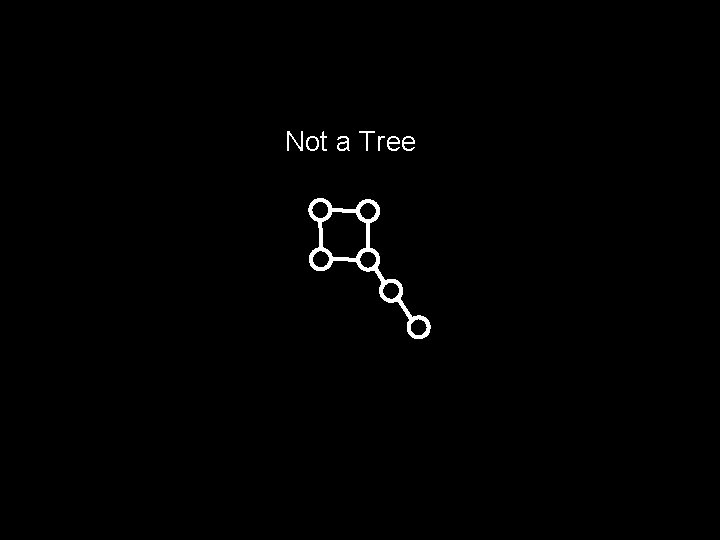Not a TreeTreeHow Many n-Node Trees? 1: 2: 3: 4: 5:Notation In this lecture: n will denote the number of nodes in a graph e will denote the number of edges in a graph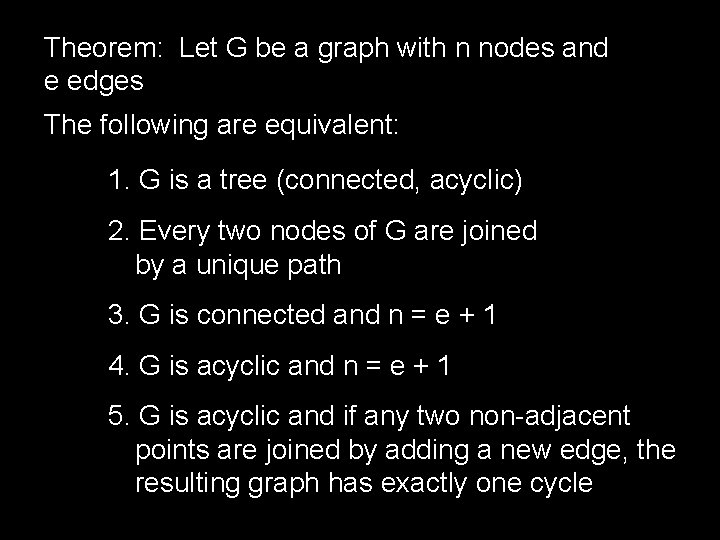Theorem: Let G be a graph with n nodes and e edges The following are equivalent: 1. G is a tree (connected, acyclic) 2. Every two nodes of G are joined by a unique path 3. G is connected and n = e + 1 4. G is acyclic and n = e + 1 5. G is acyclic and if any two non-adjacent points are joined by adding a new edge, the resulting graph has exactly one cycleTo prove this, it suffices to show 1 2 3 4 5 11 2 1. G is a tree (connected, acyclic) 2. Every two nodes of G are joined by a unique path Proof: (by contradiction) Assume G is a tree that has two nodes connected by two different paths: Then there exists a cycle!2 3 2. Every two nodes of G are joined by a unique path 3. G is connected and n = e + 1 Proof: (by induction) Assume true for every graph with < n nodes Let G have n nodes and let x and y be adjacent G 1 x y G 2 Let n 1, e 1 be number of nodes and edges in G 1 Then n = n 1 + n 2 = e 1 + e 2 + 2 = e + 13 4 3. G is connected and n = e + 1 4. G is acyclic and n = e + 1 Proof: (by contradiction) Assume G is connected with n = e + 1, and G has a cycle containing k nodes Note that the cycle has k nodes and k edges Starting from cycle, add other nodes and edges until you cover the whole graph Number of edges in the graph will be at least nCorollary: Every nontrivial tree has at least two endpoints (points of degree 1) Proof: Assume all but one of the points in the tree have degree at least 2 In any graph, sum of the degrees = 2 e Then the total number of edges in the tree is at least (2 n-1)/2 = n - 1/2 > n - 1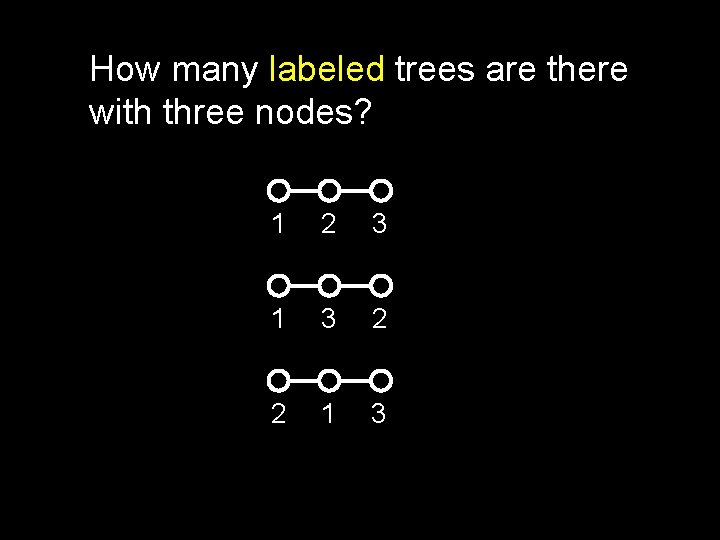How many labeled trees are there with three nodes? 1 2 3 1 3 2 2 1 3How many labeled trees are there with four nodes? a c b d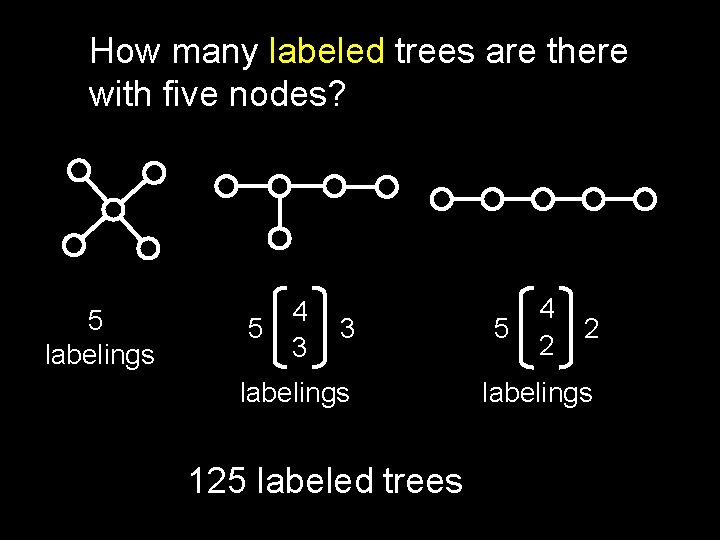How many labeled trees are there with five nodes? 5 labelings 5 4 3 3 labelings 125 labeled trees 5 4 2 2 labelings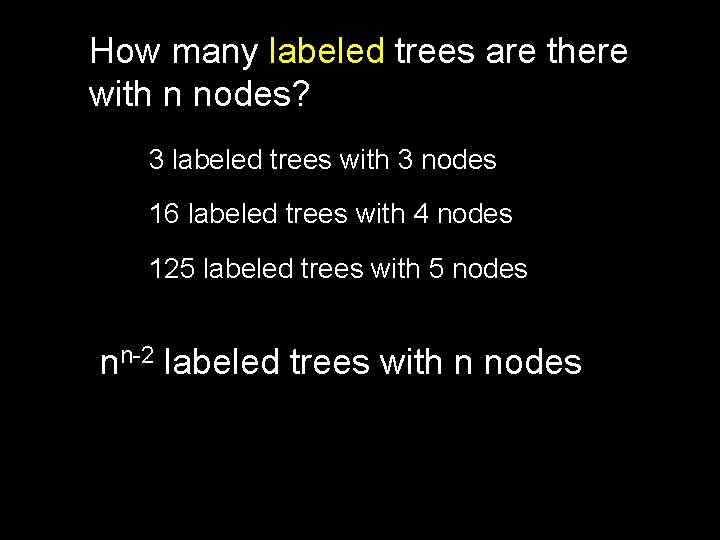How many labeled trees are there with n nodes? 3 labeled trees with 3 nodes 16 labeled trees with 4 nodes 125 labeled trees with 5 nodes nn-2 labeled trees with n nodesCayley’s Formula The number of labeled trees on n nodes is nn-2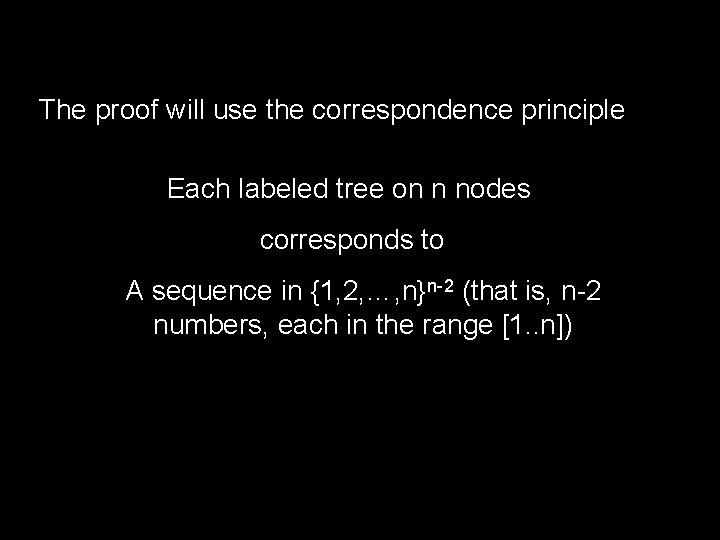The proof will use the correspondence principle Each labeled tree on n nodes corresponds to A sequence in {1, 2, …, n}n-2 (that is, n-2 numbers, each in the range [1. . n])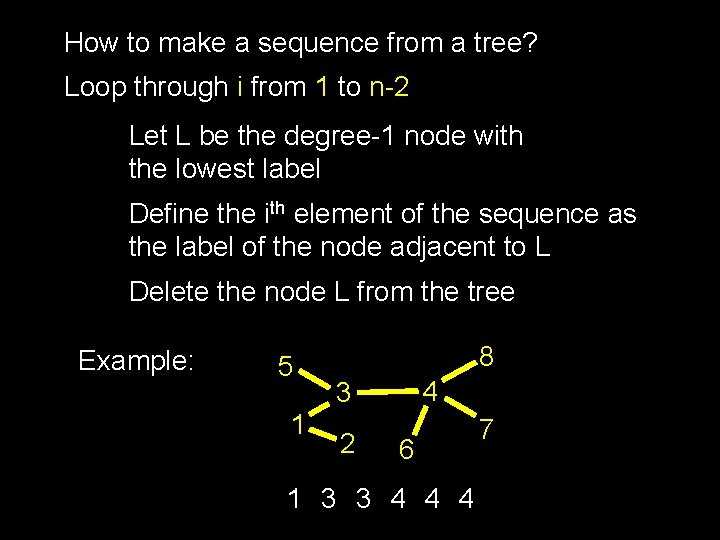How to make a sequence from a tree? Loop through i from 1 to n-2 Let L be the degree-1 node with the lowest label Define the ith element of the sequence as the label of the node adjacent to L Delete the node L from the tree Example: 5 1 8 4 3 2 6 1 3 3 4 4 4 7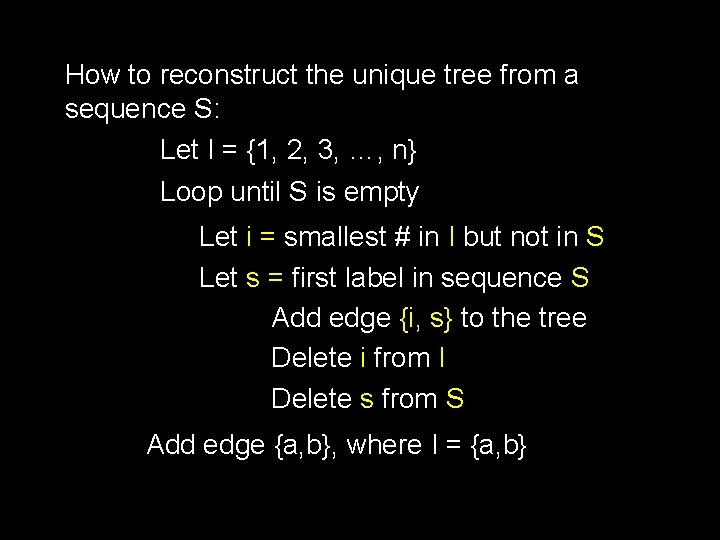How to reconstruct the unique tree from a sequence S: Let I = {1, 2, 3, …, n} Loop until S is empty Let i = smallest # in I but not in S Let s = first label in sequence S Add edge {i, s} to the tree Delete i from I Delete s from S Add edge {a, b}, where I = {a, b}S: I: 1 3 3 4 4 4 1 2 3 4 5 6 7 8 5 1 8 4 3 2 6 7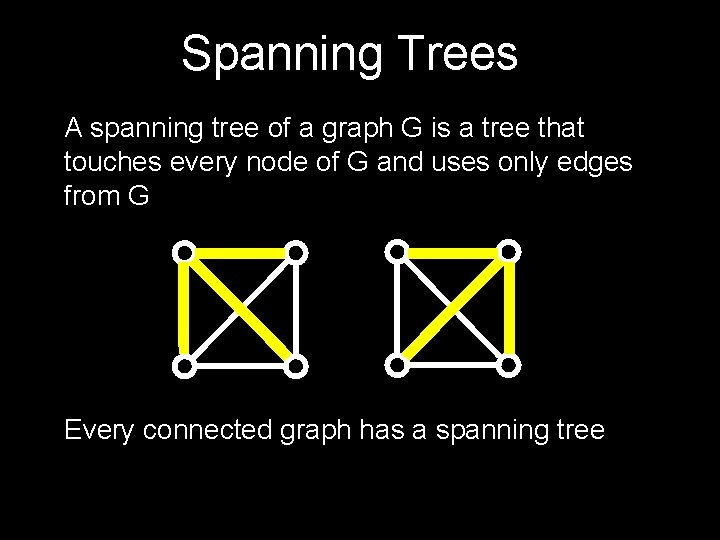Spanning Trees A spanning tree of a graph G is a tree that touches every node of G and uses only edges from G Every connected graph has a spanning tree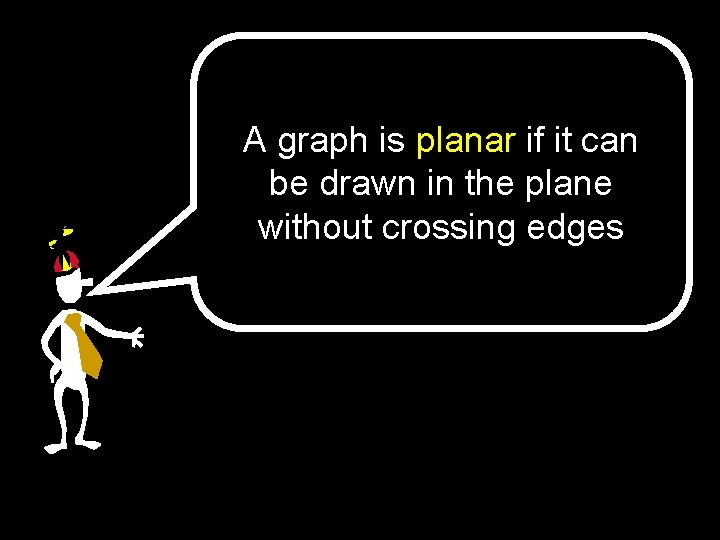A graph is planar if it can be drawn in the plane without crossing edgesExamples of Planar Graphs =Faces A planar graph splits the plane into disjoint faces 4 facesEuler’s Formula If G is a connected planar graph with n vertices, e edges and f faces, then n–e+f=2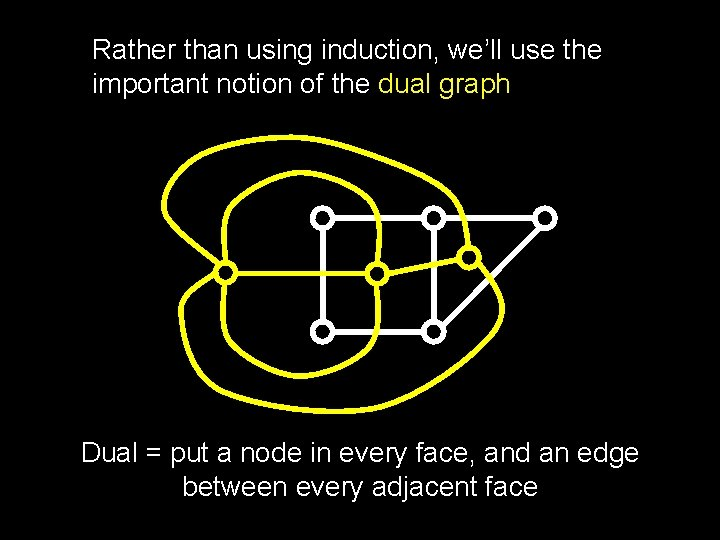Rather than using induction, we’ll use the important notion of the dual graph Dual = put a node in every face, and an edge between every adjacent faceLet G* be the dual graph of G Let T be a spanning tree of G Let T* be the graph where there is an edge in dual graph for each edge in G – T Then T* is a spanning tree for G* n = e. T + 1 f = e. T* + 1 n + f = e. T + e. T* + 2 =e+2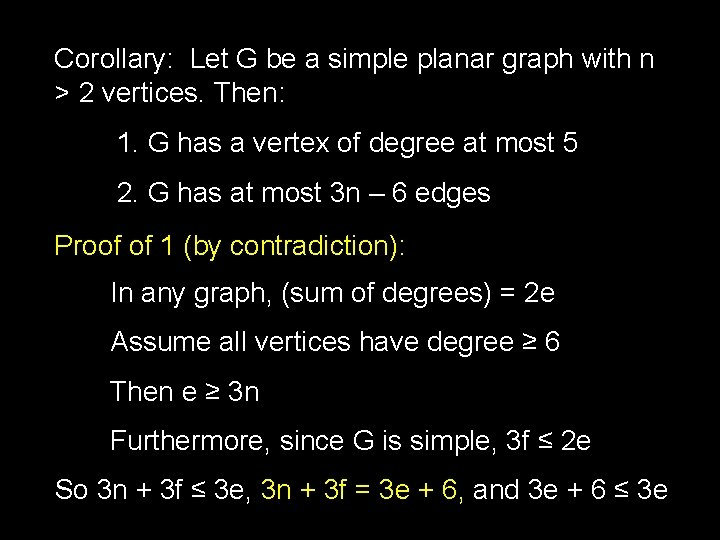Corollary: Let G be a simple planar graph with n > 2 vertices. Then: 1. G has a vertex of degree at most 5 2. G has at most 3 n – 6 edges Proof of 1 (by contradiction): In any graph, (sum of degrees) = 2 e Assume all vertices have degree ≥ 6 Then e ≥ 3 n Furthermore, since G is simple, 3 f ≤ 2 e So 3 n + 3 f ≤ 3 e, 3 n + 3 f = 3 e + 6, and 3 e + 6 ≤ 3 eGraph Coloring A coloring of a graph is an assignment of a color to each vertex such that no neighboring vertices have the same color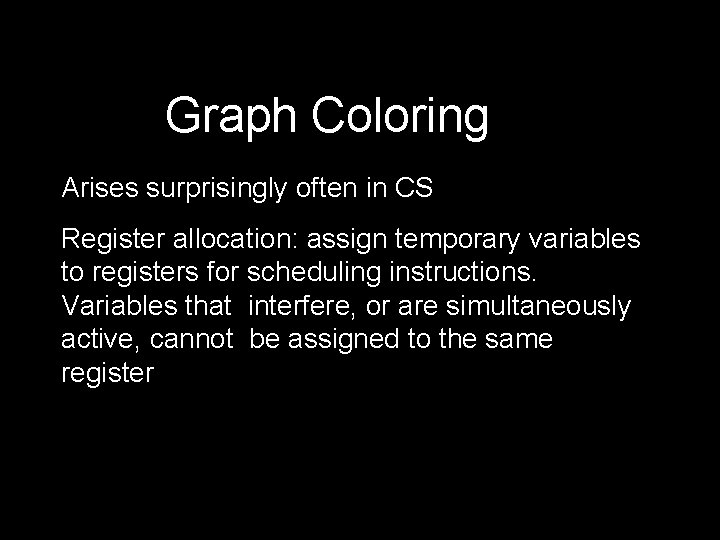Graph Coloring Arises surprisingly often in CS Register allocation: assign temporary variables to registers for scheduling instructions. Variables that interfere, or are simultaneously active, cannot be assigned to the same registerTheorem: Every planar graph can be 6 -colored Proof Sketch (by induction): Assume every planar graph with less than n vertices can be 6 -colored Assume G has n vertices Since G is planar, it has some node v with degree at most 5 Remove v and color by Induction HypothesisNot too difficult to give an inductive proof of 5 colorability, using same fact that some vertex has degree ≤ 5 4 -color theorem remains challenging!Implementing Graphs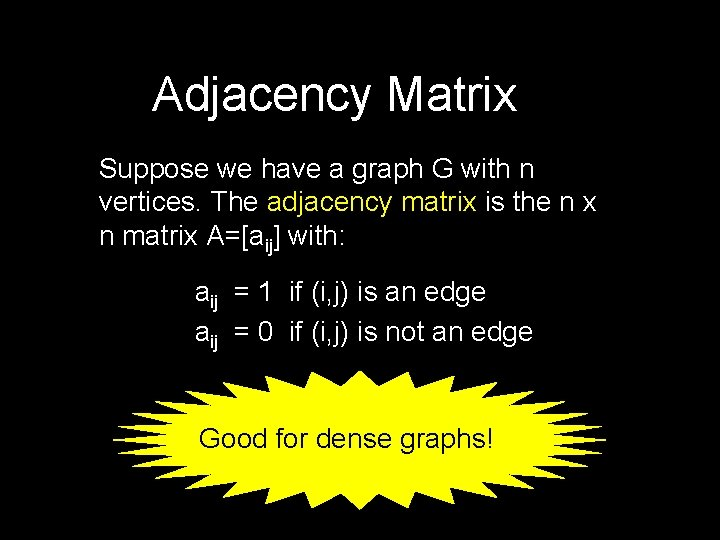Adjacency Matrix Suppose we have a graph G with n vertices. The adjacency matrix is the n x n matrix A=[aij] with: aij = 1 if (i, j) is an edge aij = 0 if (i, j) is not an edge Good for dense graphs!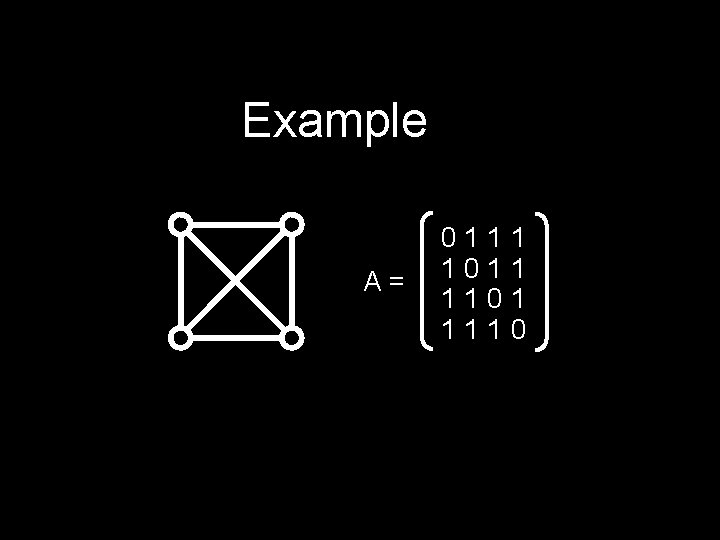Example A= 0111 1011 1101 1110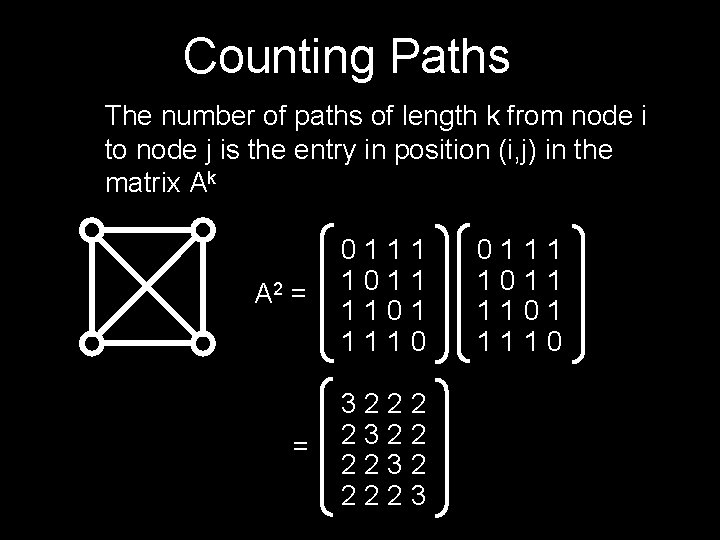Counting Paths The number of paths of length k from node i to node j is the entry in position (i, j) in the matrix Ak A 2 = 0111 1011 1101 1110 = 3222 2322 2232 2223 0111 1011 1101 1110Adjacency List Suppose we have a graph G with n vertices. The adjacency list is the list that contains all the nodes that each node is adjacent to Good for sparse graphs!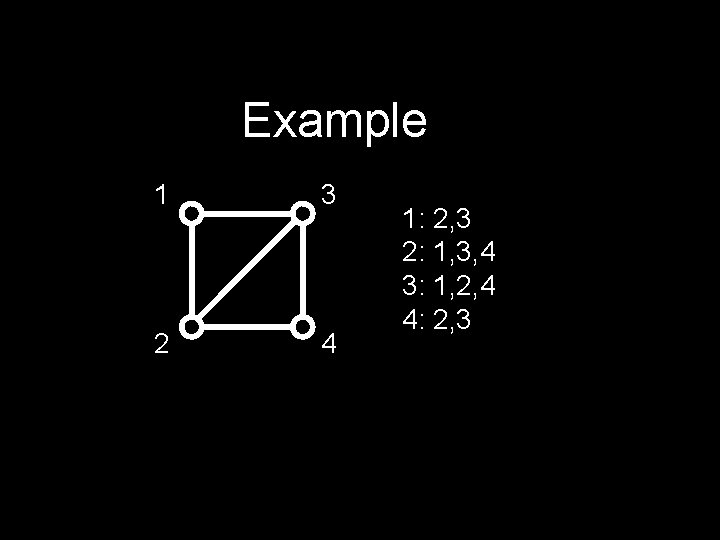Example 1 3 2 4 1: 2, 3 2: 1, 3, 4 3: 1, 2, 4 4: 2, 3Trees • Counting Trees • Different Characterizations Planar Graphs • Definition • Euler’s Theorem • Coloring Planar Graphs Here’s What You Need to Know… Adjacency Matrix and List • Definition • Useful for counting